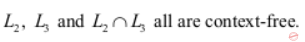October 4, 2023
October 4, 2023
October 4, 2023
###### Operating-Systems
October 4, 2023
 Question 15
Consider the following languages:A L 1 is not context-free but L 2 and L 3 are deterministic context-free. B Neither L 1 nor L 2 is context-free. CD Neither L 1 nor its complement is context-free.
Question 15 Explanation:
L1=ww is a well-known CSL (non-CFL) and its complement is CFL.
L2={an bn cm | m,n >=0} this contains only one comparison (number of a’s = number of b’s) so this is DCFL.
L3={am bn cn | m,n >=0} this contains only one comparison (number of b’s = number of c’s) so this is DCFL.
Intersection of L2 and L3 will have (number of a’s= number of b’s = number of c’s) i.e., {an bn cn | n >=0} so this is CSL (non-CFL).
So A is a true statement and rest all are false statements.
Question 15 Explanation:
L1=ww is a well-known CSL (non-CFL) and its complement is CFL.
L2={an bn cm | m,n >=0} this contains only one comparison (number of a’s = number of b’s) so this is DCFL.
L3={am bn cn | m,n >=0} this contains only one comparison (number of b’s = number of c’s) so this is DCFL.
Intersection of L2 and L3 will have (number of a’s= number of b’s = number of c’s) i.e., {an bn cn | n >=0} so this is CSL (non-CFL).
So A is a true statement and rest all are false statements.